#Function Repository Resource:

# ExpressionBag

A data structure meant for accumulating items efficiently

Contributed by: Richard Hennigan (Wolfram Research)
 ResourceFunction["ExpressionBag"]["id"] retrieves the expression bag specified by "id". ResourceFunction["ExpressionBag"][list] creates an expression bag using list as the initial data. ResourceFunction["ExpressionBag"][…][prop] returns the specified property of the bag. ResourceFunction["ExpressionBag"][…]["Add",expr] adds expr to the bag. ResourceFunction["ExpressionBag"][…]["Part",part] gets the expression specified by part from the bag.

## Details and Options

In ResourceFunction["ExpressionBag"]["id"], the "id" can be the name or UUID of an expression bag.
The following operations on an expression bag are supported:
 Length [ bag ] the number of items contained in the bag Append [ bag , expr ] adds expr to the bag bag [ [ part ] ] gets items from the bag specified by Part specification part Get [ bag ] gets all items from the bag Information [ bag ] returns a summary of information about the bag DeleteObject [ bag ] permanently delete bag
ResourceFunction["ExpressionBag"] is a single interface to the internal functions Internal`Bag, Internal`StuffBag, Internal`BagLength and Internal`BagPart.
Using ResourceFunction["ExpressionBag"][]["Add",expr] is a more efficient option for situations where one might consider using constructs such as AppendTo[list,expr] as a way of accumulating data.

## Examples

### Basic Examples

Create an empty expression bag:

 In:=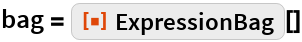Out=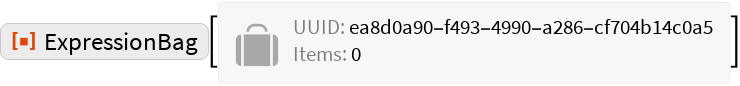Add values to the expression bag:

 In:=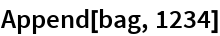Out=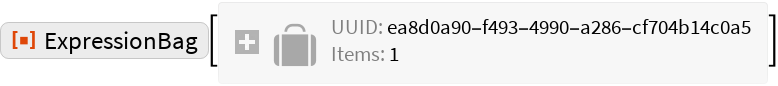In:=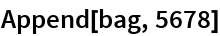Out=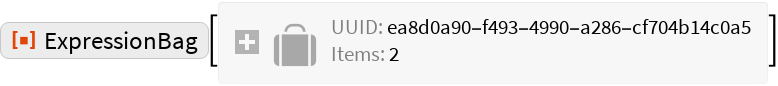Retrieve all values from the bag:

 In:=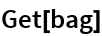Out=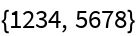Create an expression bag from some initial data:

 In:=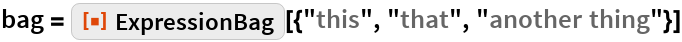Out=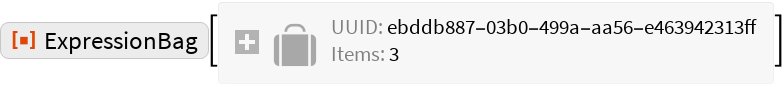Retrieve parts of the expression bag:

 In:=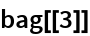Out=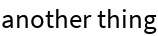In:=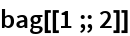Out=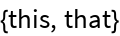Create a named expression bag:

 In:=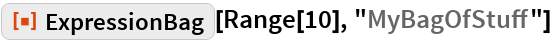Out=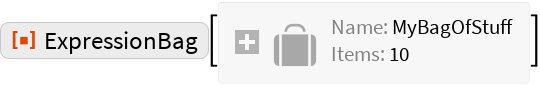Reference the bag by name:

 In:=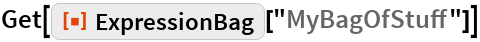Out=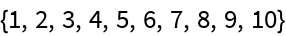### Scope

Get information about an expression bag:

 In:=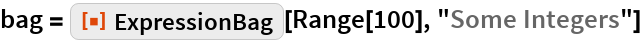Out=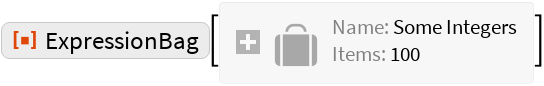In:=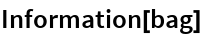Out=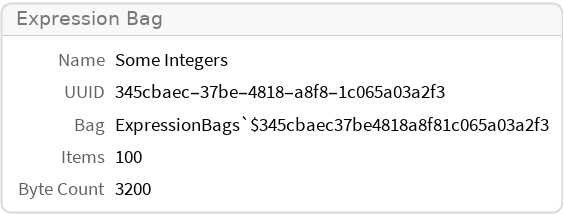Delete an expression bag:

 In:=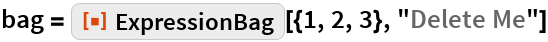Out=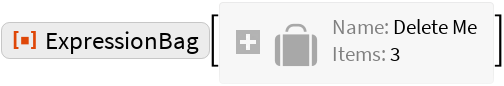In:=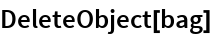The expression bag can no longer be referenced by name:

 In:=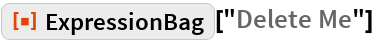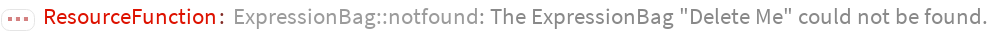Out=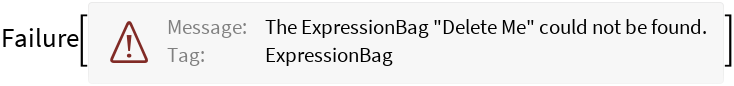Get all expression bags in the current session:

 In:=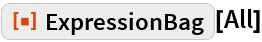Out=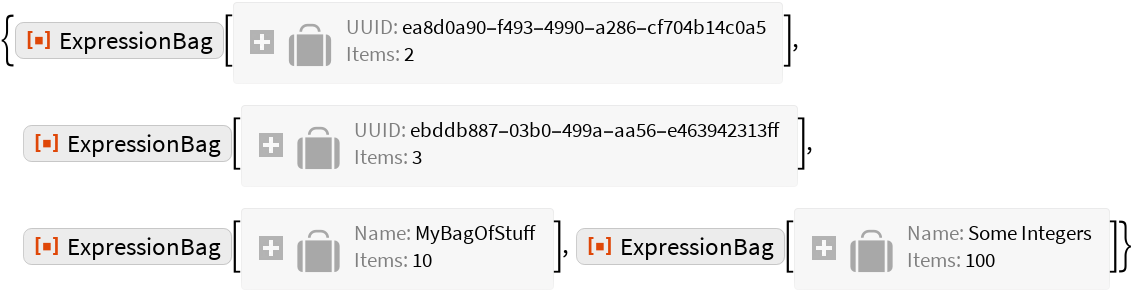Delete them:

 In:=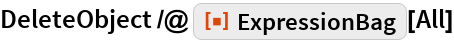Out=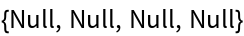### Applications

Keep a running history of inputs in the current session:

 In:=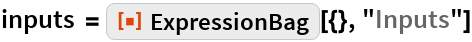Out=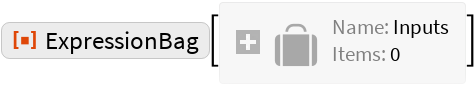In:=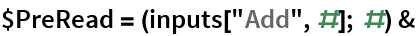Out=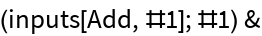Evaluate some inputs:

 In:=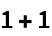Out=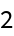In:=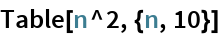Out=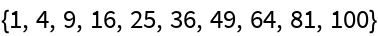In:=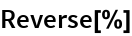Out=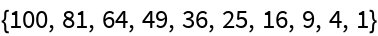Get the list of inputs entered so far:

 In:=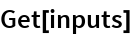Out=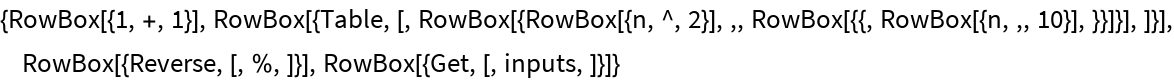Create a notebook showing input history:

 In:=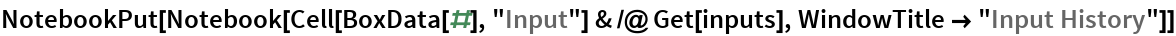Out=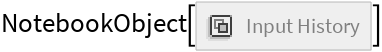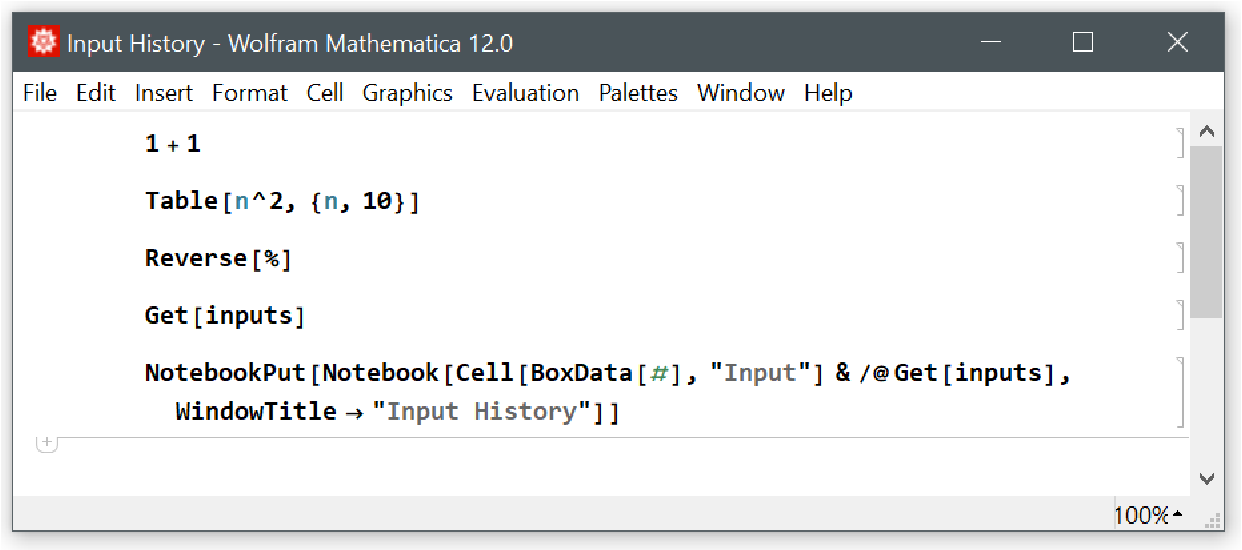In:=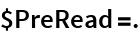### Properties and Relations

An expression bag can be used as a collection that allows efficient insertion of items:

 In:=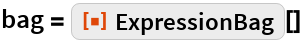Out=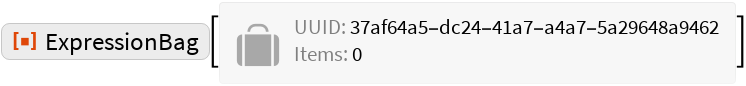In:=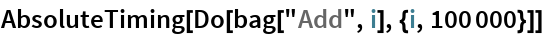Out=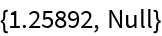Compare to using AppendTo and a List:

 In:=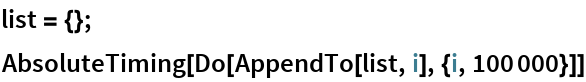Out=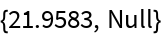The results are equivalent:

 In:=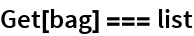Out=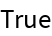The insertion time for an expression bag is not affected by the item count:

 In:=Out=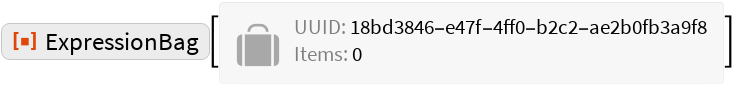In:=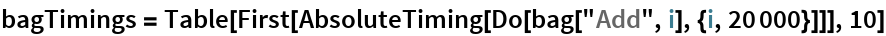Out=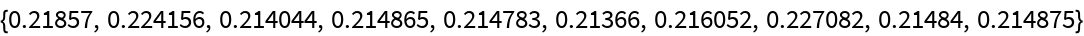Compare to using AppendTo and List:

 In:=Out=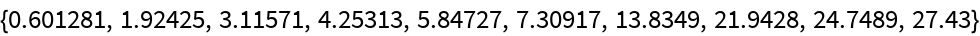In:=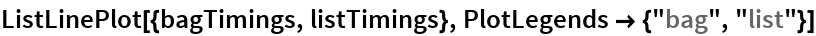Out=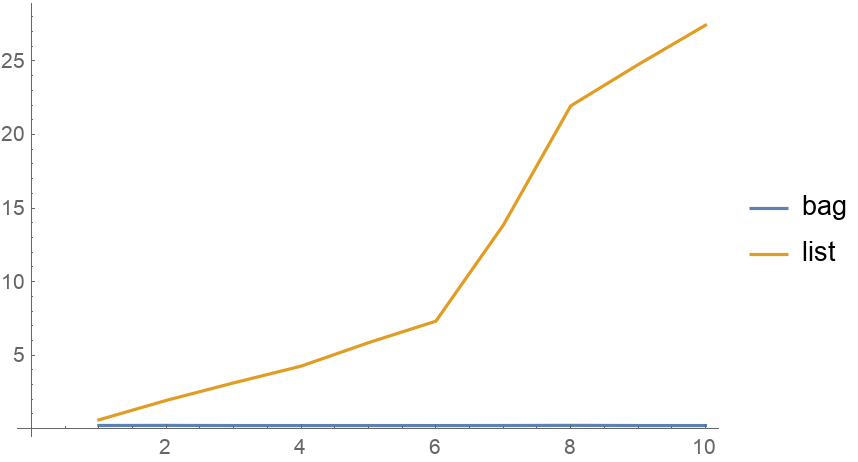## Requirements

Wolfram Language 11.3 (March 2018) or above